# Errata

1. Page 33. Third displayed equation and text should be:As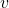and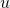are independent and normal,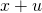is normal with mean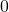2. Page 33. Fourth displayed equation, and sentence that follows should read: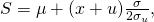so that the linear sensitivity parameter is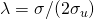.

4. Page 37: the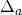inin the denominator should be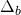. This is the right equation5. Pages 110-111. The equations below (5.21) onwards should be
•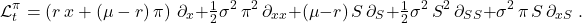•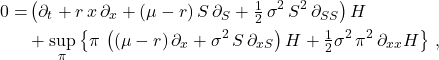••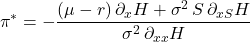•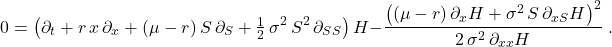6. Page 111, the inequality in point (ii) should be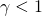NOT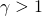. For, there is an upper bound on wealth, rather than a lower bound.
7. In the exercises of Chapter 6 the liquidation penalty has the same typo in
three places. The penalty is not ‘minus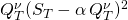it should be ‘plus‘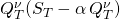The typos appear in first displayed equation in E.6.1, and equations (6.41) and (6.47)
8. Page 175. There is a minus sign missing in equation (7.22) . The equation should be: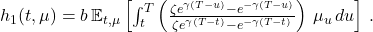9. Equations (A.8), (A.11), (A.17), and (A.18) are all missing the term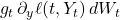. This change does NOT, however, alter the corresponding differential operators, as this term represents a martingale increment.
Feel free to contact us if you find any. If you can, please use the following format
Section:
Page:
Equation/figure/table number:
The error is:
Also, we prefer it if you send the maths in LaTeX format